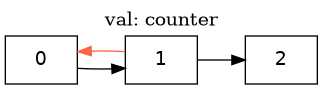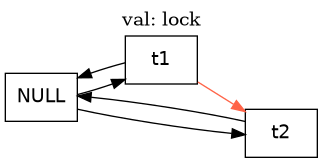# Action Properties¶

## Action Properties¶

In the last chapter I said that all invariants are safety properties, but not all safety properties are invariants. Outside invariants, the biggest class of safety properties are “action properties”, which are restrictions on how the system is allowed to change.

Let’s play a bit more with the threads spec:

---- MODULE threads ----
EXTENDS TLC, Integers
CONSTANT NULL

variables
counter = 0;
lock = NULL;

define
end define;

variables tmp = 0;
begin
GetLock:
await lock = NULL;
lock := self;

GetCounter:
tmp := counter;

IncCounter:
counter := tmp + 1;

ReleaseLock:
lock := NULL;
end process;
end algorithm; *)
====

19 states / 17 distinct spec

Here are a couple of restrictions on the way the spec should be allowed to change:

• counter should only increase.

• If a thread holds the lock, it cannot go to another thread without being unlocked first.

Here’s how we write the first as an action property:

   lock = NULL;

define
+  CounterOnlyIncreases ==
+    [][counter' >= counter]_counter
end define;



Wait, what?

Don’t worry, I’ll explain this syntax in the next section. For now, let’s confirm that this actually breaks. First make a change where counter decreases:

     tmp := counter;

IncCounter:
-    counter := tmp + 1;
+    counter := tmp + IF tmp = 0 THEN 1 ELSE -1;

ReleaseLock:
lock := NULL;


Now run this with PROPERTY CounterOnlyIncreases (not as an invariant). If set up right, you should see this error:

\* some initial states
State 7:
/\ counter = 1
/\ lock = 1
/\ pc = <<"IncCounter", "Done">>
/\ tmp = <<1, 0>>

State 8:
/\ counter = 0
/\ lock = 1
/\ pc = <<"ReleaseLock", "Done">>
/\ tmp = <<1, 0>>


This doesn’t fail because we have a state where the counter is 0. That’s a totally valid state for the spec, and is in fact the starting state! It fails because counter changes from 1 to 0. It’s the fact counter decreases that’s an error.None of these states are illegal, but the transition from counter=1 to counter=0 is.¶

### Understanding the Syntax¶

On one hand, cool trick. On the other, we now have to figure out what [][counter' >= counter]_counter is supposed to mean.

It’s finally time to talk about the “actions” in “Temporal Logic of Actions”.

So remember how way back I said that strings must use double quotes? That’s because single quotes have a special role in TLA+. In any given step, x' is the value of x at the end of the step and the value x starts as in the next step. [](x' >= x), then, is “it is always true that the next value of x is larger than or equal to x”.

Tip

you can use primed operators in the trace explorer. It’ll show you the value of the expression in the next step.

But that’s not (yet) a valid TLA+ property. Consider the slightly different property [](x' = x + 1): “x always increases by exactly one”. What happens if we insert a stutter step? Then x doesn’t change at all, which means that the property is false. But by the definition of TLA+, we can always insert a stutter step anywhere. So this property is trivially false. The more interesting property we actually wanted to check was [](x' # x => x' + 1). Alternatively, we can write this as x' > x \/ UNCHANGED x.

As yet more syntactic sugar, we can write [](x' = x + 1 \/ UNCHANGED x) as [][x' = x + 1]_x. This is called a box action formula. Box action formulas have a special role in TLA+, as we’ll see in the next chapter. TLC can only check action properties that are box action formulas.

Tip

The underscory bit (_) means that we could have written the property as [][counter' > counter]_counter. Expanding all the steps:

1. [counter' > counter]_counter

2. counter' > counter \/ UNCHANGED counter

3. counter' > counter \/ counter' = counter

4. counter' >= counter

But in general, you shouldn’t rely on that aspect of []_x for your property. If it’s okay for counter to stay the same, make that explicit.

### More Action Properties¶

Let’s add another property that “the lock can’t go straight from one thread to another”:

 define
CounterOnlyIncreases ==
[][counter' >= counter]_counter
+
+  LockCantBeStolen ==
+    [][lock # NULL => lock' = NULL]_lock
end define;

@@ -27,7 +30,7 @@
tmp := counter;

IncCounter:
-    counter := tmp + IF tmp = 0 THEN 1 ELSE -1;
+    counter := tmp + 1;

ReleaseLock:
lock := NULL;


And now we’ll make a change that breaks this property:

 variables tmp = 0;
begin
GetLock:
-    await lock = NULL;
lock := self;

GetCounter:


Running with PROPERTY LockCantBeStolen shows this fail.Another way we could have written the property:


LockCantBeStolen ==
[][lock # NULL => lock' = NULL]_lock
+
+  LockNullBeforeAcquired ==
+    [][lock' # NULL => lock = NULL]_lock
end define;



You can use helper actions in your action properties, so we could do something like

BecomesNull(x) == x' = NULL

LockCantBeStolen ==
[][lock # NULL => BecomesNull(lock)]_lock


### Quantified Action Properties¶

I mentioned earlier that TLC can only check top-level action properties. This can make some things a little awkward. Let’s write a quick spec with several independent counters:

---- MODULE counters ----
EXTENDS Integers

Counters == {1, 2}
(* --algorithm counters
variables
values = [i \in Counters |-> 0];

define
end define;

macro increment() begin
values[self] := values[self] + 1;
end macro

process counter \in Counters
begin
A:
increment();
B:
increment();
end process;
end algorithm; *)
=====

14 states / 9 distinct spec

As before, we want an action property saying that the counters are monotonic. Unlike before, we have several counters we need to quantify over.

   values = [i \in Counters |-> 0];

define
+  CounterOnlyIncreases ==
+      \A c \in Counters:
+        [][values[c]' >= values[c]]_values[c]
end define;

macro increment() begin


Unfortunately, TLC can’t check this, due to limitations of the model checker.

[] followed by action not of form [A]_v.

(The error is a little confusing, but it happens whenever we put our action property inside a quantifier).

What we can do in this case is pull the quantifier inside the action property. It turns out that [] commutes with \A! In other words, any equation written \A x: []P(x) is equivalent to the formula [](\A x: P(x)).


define
CounterOnlyIncreases ==
+    [][
\A c \in Counters:
-        [][values[c]' >= values[c]]_values[c]
+        values[c]' >= values[c]
+      ]_values
end define;

macro increment() begin


## Using Action Properties¶

Most of the specs I write have more invariants than action properties and more action properties than liveness properties. But liveness properties are arguably more “important” than action properties, as every spec needs at least one. Action props are powerful but optional.

Nonetheless, I love using action properties. They give you an incredible amount of flexibility for defining new properties.

## Summary¶

• Action properties are properties on transitions of a system, and are checked as temporal properties.

• x' is the value of x in the next state. Operators with primes in them are called Actions.

• [P]_x means P \/ UNCHANGED x.